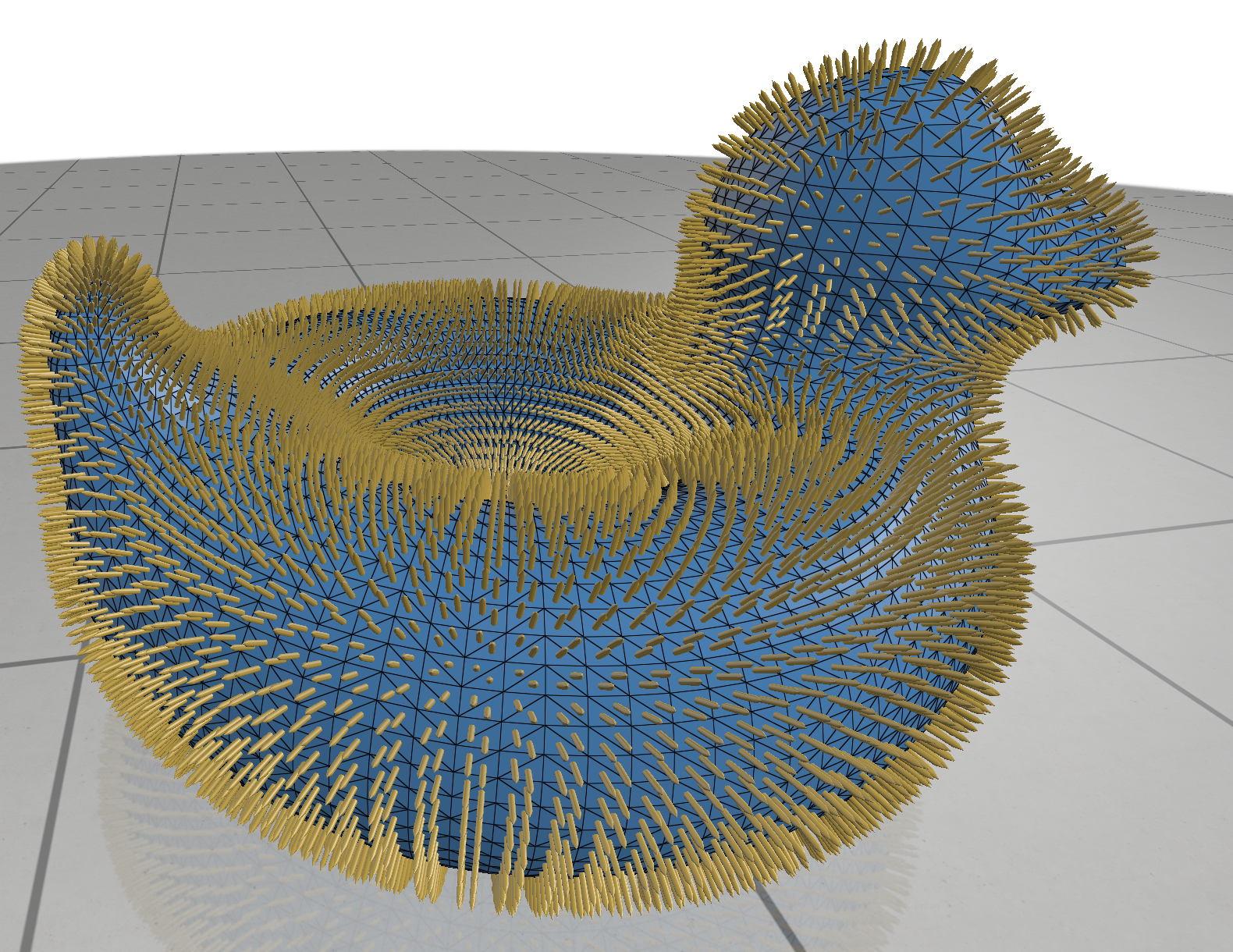# Vector Quantities

Visualize vector-valued data at the elements of a surface mesh.Example:

import numpy as np
import polyscope as ps
ps.init()

# register a surface mesh
N_vert = 100
N_face = 250
vertices = np.random.rand(N_vert, 3) # (V,3) vertex position array
faces = np.random.randint(0, N_vert, size=(N_face,3)) # (F,3) array of indices
# for triangular faces

ps_mesh = ps.register_surface_mesh("my mesh", vertices, faces)

# visualize some random vectors per vertex
vecs_vert = np.random.rand(N_vert, 3)

# set radius/length/color of the vectors
length=0.005, color=(0.2, 0.5, 0.5))

# ambient vectors don't get auto-scaled, useful e.g. when representing offsets in 3D space

# view the mesh with all of these quantities
ps.show()


SurfaceMesh.add_vector_quantity(name, values, defined_on='vertices', enabled=None, vectortype="standard", length=None, radius=None, color=None)

Add a vector quantity to the mesh.

• name string, a name for the quantity
• values an Nx3 numpy array, vectors at vertices/faces (or Nx2 for 2D data)
• defined_on string, one of vertices or faces, is this data a vector per-vertex or a vector per-face?

• enabled boolean, whether the quantity is initially enabled
• vectortype, one of standard or ambient. Ambient vectors don’t get auto-scaled, and thus are good for representing values in absolute 3D world coordinates
• length float, a (relative) length for the vectors
• radius float, a (relative) radius for the vectors
• color 3-tuple, color for the vectors

if not specified, these optional parameters will assume a reasonable default value, or a persistent value if previously set.

### Tangent vectors

Tangent vectors lie flat against the surface of the mesh. They are expressed as 2D vectors with X-Y coordinates in some basis frame at each mesh element.

#### Specifying the tangent basis

Tangent vectors are defined with respect to a coordinate frame at each vertex (resp., face). Before adding any tangent vector quantities, you probably need to tell Polyscope what this coordinate frame looks like. To do so, pass an array of the x-axis vectors (in 3D) for each mesh element.

SurfaceMesh.set_vertex_tangent_basisX(vectors)

Specify the tangent coordinate system at vertices, by giving the direction of the x-axis of the basis.

• vectors is an Vx3 (or Vx2 for 2D) array of one 3D vector at each vertex, giving the direction of the x-axis of the basis. The rest of the basis will be computed from the normal.
SurfaceMesh.set_face_tangent_basisX(vectors)

Specify the tangent coordinate system at faces, by giving the direction of the x-axis of the basis.

• vectors is an Fx3 (or Fx2 for 2D) array of one 3D vector at each face, giving the direction of the x-axis of the basis. The rest of the basis will be computed from the normal.

In these function names, intrinsic vector is a fancy synonym for tangent vector, which indicates that the vectors lie in the surface itself, not the containing 3D space.

SurfaceMesh.add_intrinsic_vector_quantity(name, values, n_sym=1, defined_on='vertices', enabled=None, vectortype="standard", length=None, radius=None, color=None, ribbon=None)

Add a vector quantity to the mesh. Remember to specify your tangent basis first!

• name string, a name for the quantity
• values an Nx2 numpy array, of tangent vectors at vertices/faces
• n_sym is a symmetry order for visualizing line fields (n = 2) and cross fields (n = 4), etc. If it is set to a non-1 value, n distinct vectors will be displayed at each element. This function presumes a “power”-representation for symmetric fields, which the inputs result from raising symmetric vectors to the n’th power.
• defined_on string, one of vertices or faces, is this data a vector per-vertex or a vector per-face?

• enabled boolean, whether the quantity is initially enabled
• vectortype, one of standard or ambient. Ambient vectors don’t get auto-scaled, and thus are good for representing values in absolute 3D world coordinates
• length float, a (relative) length for the vectors
• radius float, a (relative) radius for the vectors
• color 3-tuple, color for the vectors
• ribbon boolean, if true show the traced ribbon visualization

if not specified, these optional parameters will assume a reasonable default value, or a persistent value if previously set.

### One forms

One forms are tangent vector-like quantities represented as integrated scalars along edges. They commonly arise, for example, as a gradient which is difference of scalar values at vertices.

SurfaceMesh.add_one_form_vector_quantity(name, values, orientations, enabled=None, length=None, radius=None, color=None, ribbon=None)

Add a one-form vector quantity to the mesh. Remember, before passing edge-valued data, be sure your indexing convention matches what Polyscope expects.

• name string, a name for the quantity
• values a length n_edges numpy float array, integrated 1-form values at edges
• orientations a length n_edges numpy boolean array. 1-forms are defined with respect to an orientation of edges, so you need to tell Polyscope which direction your edges point in. These booleans should be true if the edge points from the lower indexed adjacent vertex to the higher-indexed vertex, and false otherwise.

• enabled boolean, whether the quantity is initially enabled
• length float, a (relative) length for the vectors
• radius float, a (relative) radius for the vectors
• color 3-tuple, color for the vectors
• ribbon boolean, if true show the traced ribbon visualization